Select Page

# Application of Derivatives MCQ CBSE Maths 12 Science Answers in English

Application of Derivatives MCQ CBSE Maths 12 Science Answers in English to enable students to get Answers in a narrative video format for the specific question.

Expert Teacher provides Application of Derivatives MCQ CBSE Maths 12 Science Answers through Video Answers in English language. This video solution will be useful for students to understand how to write an answer in exam in order to score more marks. This teacher uses a narrative style for a question from Application of Derivatives not only to explain the proper method of answering question, but deriving right answer too.

Please find the question below and view the Answer in a narrative video format.

Question:

Answer Video in English:

You can select video Answers from other languages also. Please check Answers in ( Hindi )

## Similar Questions from CBSE, 12th Science, Maths, Application of Derivatives

Question 1 : Find the maximum value of in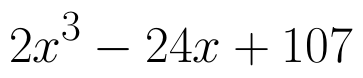the interval [1,3]. find the maximum value of the same function in [-3,-1]. (View Answer Video)

Question 2 : The points on the curve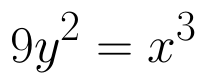, where the normal to the curve makes equal intercepts with the axes are, (View Answer Video)

Question 3 : On which of the following intervals in the function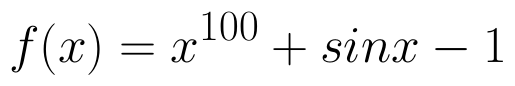strictly decreasing? (View Answer Video)

Question 4 : Find the maximum value of inthe interval [1,3]. find the maximum value of the same function in [-3,-1]. (View Answer Video)

Question 5 : Which of the following functions are strictly decreasing on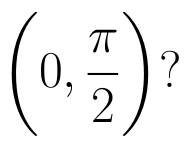(View Answer Video)

### Differential Equations

Question 1 : If x cos(a + y) = cos y, then prove that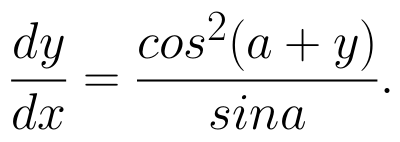Hence show that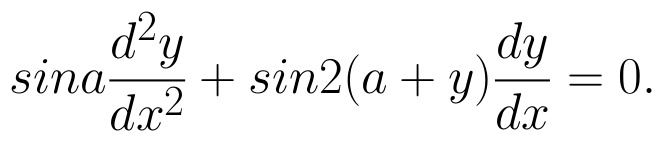(View Answer Video)

Question 2 : Solve the differential equation: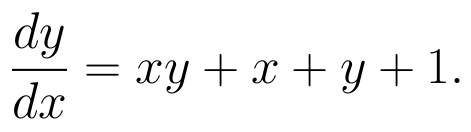(View Answer Video)

Question 3 : Solve the differential equation: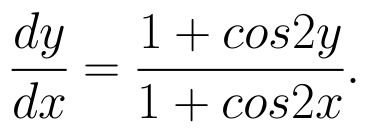(View Answer Video)

Question 4 : Write the degree of the differential equation :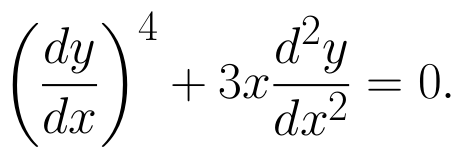(View Answer Video)

Question 5 : Find the solution of the differential equation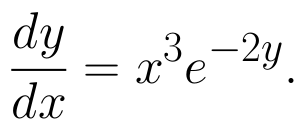(View Answer Video)

### Relations and Functions

Question 1 : A function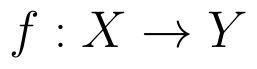is bijective if and only if, (View Answer Video)

Question 2 : Let * be the binary operation on N given by a * b = LCM of a and b. Find 20 * 16. (View Answer Video)

Question 3 : Let * be the binary operation on N given by a * b = LCM of a and b. Find the identity of * in N. (View Answer Video)

Question 4 : Let A = {1, 2, 3, 4} and let R = {(2, 2), (3, 3), (4, 4), (1, 2)} be a relation on A. Then, R is, (View Answer Video)

Question 5 :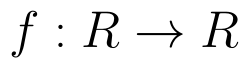be  defined as f(x) =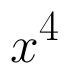(View Answer Video)

### Continuity and Differentiability

Question 1 : Differentiate the function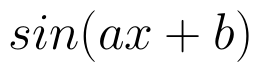with respect to x. (View Answer Video)

Question 2 : Differentiate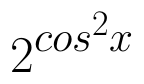w.r.t.x. (View Answer Video)

Question 3 : If x and y are connected parametrically by the equation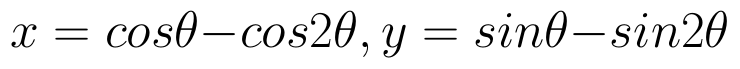, without eliminating the parameter, find. (View Answer Video)

Question 4 :  Find the second order derivative of the function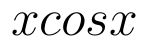. (View Answer Video)

Question 5 : Findfor the function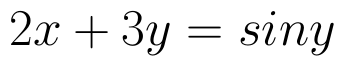. (View Answer Video)